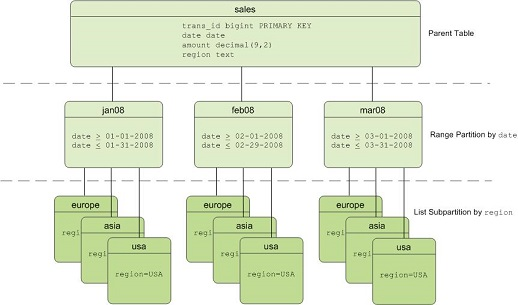If a table is partitioned, the system may scan only the partitions that meet specified query conditions, instead of scanning the full table. This improves query performance.

## Use partitioned tables

AnalyticDB for PostgreSQL supports the range, list, and multi-level partitioned tables. The following figure shows a multi-level partitioned table that has date-based range partitions and region-based list partitions.Scenarios

Take note of the following items when you decide whether to partition a table:

• Data volumes: We recommend that you use partitioning on tables that contain large volumes of data, such as a fact table that has 10 million data rows. The standard varies with your experience and the current query performance.
• Partition columns: If a table has large volumes of data and an appropriate partition column such as day or month, you can use partitioning on this table.
• Data lifecycle: Typically, data must be managed in data warehouses based on their lifecycles. You can use partitioned tables to facilitate data management such as the deletion of old data.
• Query statements: When a table is partitioned, its query performance can be improved only when its partition column is contained in query statements. If no partition columns are contained in the query statements executed on the partitioned table, it may take longer for the queries to complete because all partitions are scanned.

Create range partitioned tables

You can have AnalyticDB for PostgreSQL automatically generate partitions by assigning a START value, an END value, and an EVERY clause that defines the partition increment value. By default, START values are inclusive and END values are exclusive. Example:

``````CREATE TABLE sales (id int, date date, amt decimal(10,2))
DISTRIBUTED BY (id)
PARTITION BY RANGE (date)
( START (date '2016-01-01') INCLUSIVE
END (date '2017-01-01') EXCLUSIVE
EVERY (INTERVAL '1 day') );``````

You can create a range partitioned table that uses a column of a numeric data type as the partition key. Example:

``````CREATE TABLE rank (id int, rank int, year int, gender char(1), count int)
DISTRIBUTED BY (id)
PARTITION BY RANGE (year)
( START (2006) END (2016) EVERY (1),
DEFAULT PARTITION extra ); ``````

Create list partitioned tables

A list partitioned table can use a data type column that allows equality comparisons as a partition key. For list partitions, you must declare a partition specification for every partition (list value) that you want to create. Example:

``````CREATE TABLE rank (id int, rank int, year int, gender
char(1), count int )
DISTRIBUTED BY (id)
PARTITION BY LIST (gender)
( PARTITION girls VALUES ('F'),
PARTITION boys VALUES ('M'),
DEFAULT PARTITION other );``````

Create multi-level partitioned tables

You can create a table that has multi-level partitions. The following example shows a three-level partitioning design. Data in range partitions is partitioned by month, and data in list partitions is partitioned by region.

``````CREATE TABLE sales
(id int, year int, month int, day int, region text)
DISTRIBUTED BY (id)
PARTITION BY RANGE (month)
SUBPARTITION BY LIST (region)
SUBPARTITION TEMPLATE (
SUBPARTITION usa VALUES ('usa'),
SUBPARTITION europe VALUES ('europe'),
SUBPARTITION asia VALUES ('asia'),
DEFAULT SUBPARTITION other_regions)
(START (1) END (13) EVERY (1),
DEFAULT PARTITION other_months );``````

## Optimize partitioned table queries

• Partition granularity

For a table partitioned by time, the granularity can be a day, week, or month. A finer granularity results in a smaller amount of data in each partition but a larger number of partitions. The number of partitions is not measured by an absolute standard. Typically, 200 partitions are considered a large amount. A large number of partitions has a significant impact on database performance. For example, the query optimizer may take a longer time to generate execution plans, and maintenance operations such as VACUUM may slow down.

In a multi-level partitioned table, the number of partitioning files may increase sharply. For example, if a table is partitioned by month and city in a scenario that contains 24 months and 100 cities, the total number of table partitions is 2,400. Assume that the table is a column-oriented table that stores each column in a physical table. If the table contains 100 columns, the system must manage more than 100,000 files for the table. You must estimate the number of partitions that are needed when you determine the partitioning method.

• Partition pruning

AnalyticDB for PostgreSQL supports partition pruning for partitioned tables. Only required partitions are scanned based on query conditions, which improves query performance. Example:

``````explain
select * from sales
where year = 2008
and  month = 1
and  day = 3
and  region = 'usa';``````

The query conditions fall on the level-3 partition 'usa' of the level-2 partition 1 of level-1 partition 2008. Therefore, only the data in the level-3 partition 'usa' is scanned during the query. The following query plan shows that only one of the 468 level-3 partitions is read.

``````Gather Motion 4:1  (slice1; segments: 4)  (cost=0.00..431.00 rows=1 width=24)
->  Sequence  (cost=0.00..431.00 rows=1 width=24)
->  Partition Selector for sales (dynamic scan id: 1)  (cost=10.00..100.00 rows=25 width=4)
Filter: year = 2008 AND month = 1 AND region = 'usa'::text
Partitions selected:  1 (out of 468)
->  Dynamic Table Scan on sales (dynamic scan id: 1)  (cost=0.00..431.00 rows=1 width=24)
Filter: year = 2008 AND month = 1 AND day = 3 AND region = 'usa'::text``````

## Maintain partitioned tables

In partitioned tables, partitions can be managed by using various SQL statements. Examples:

• Create a partition

If a default partition exists, you cannot create partitions, but can split the default partition.

``ALTER TABLE test_partition_range ADD partition p2 start ('2017-02-01') end ('2017-02-28');``
• Delete a partition
``ALTER TABLE test_partition_range DROP partition p2;``
• Rename a partition
``ALTER TABLE test_partition_range RENAME PARTITION p2 TO Feb17;``
• Clear a partition
``ALTER TABLE test_range_partition TRUNCATE PARTITION p1;``
• Exchange a partition
``ALTER TABLE test_range_partition EXCHANGE PARTITION p2 WITH  TABLE {cos_table_name} ;``
• Split a partition
``````-- Split the p2 partition into two partitions on February 20, 2017.
ALTER TABLE test_partition_range SPLIT partition p2 at ('2017-02-20') into (partition p2, partition p3); ``````